Question Pool Area

# Area - SAMAGRA Question Pool & Answers | Class 9 English Medium

Kerala Syllabus SAMAGRA SCERT SAMAGRA Question Pool for Class 9 English Medium Maths AreaQn 1.

PQ = 7 cm, QR = 6cm, ∠Q=40. Then

a) Draw the triangle PQR.

b) Draw a triangle with the same area and with one side PR.

a) For drawing triangle   PQR

b)For

Get Free Study Materials + 1 Week Free Trial of BrainsPrep Class 9 English Medium Tuition

Qn 2.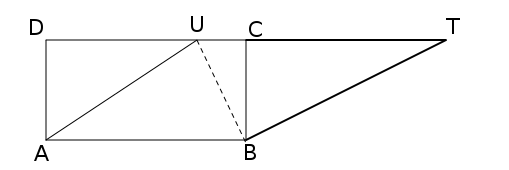In the figure ABCD is a rectangle and ABTU is a parallelogram. Then

a) If area of triangle ABU is k then what is the area of the parallelogram

b) Prove that DU = CT

Get Free Study Materials + 1 Week Free Trial of BrainsPrep Class 9 English Medium Tuition

Qn 3.

In triangle ABC, AB=6 cm, ∠A=55˚, ∠B=60˚. Draw triangle ABC. Draw an isosceles triangle with the same area.

For drawing triangle  ABC

Drawing an isosceles triangle with the same

Get Free Study Materials + 1 Week Free Trial of BrainsPrep Class 9 English Medium Tuition

Qn 4.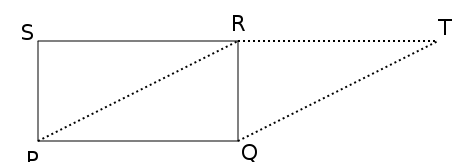Area of the rectangle PQRS is 120 sq. cm. What is the area of the parallelogram PQTR, why?

Area ofPQRS  = 120 sq.cm.

Area of triangle  PQR  = 60

Get Free Study Materials + 1 Week Free Trial of BrainsPrep Class 9 English Medium Tuition

Qn 5.
PQRS is a rectangle and triangle PQT is isosceles and PQ=8 cm. Then

a) What is the measure of ∠SPT.

b) What is the breadth of rectangle PQRS.

c) Find the area of the rectangle

d) What is the area of each triangles?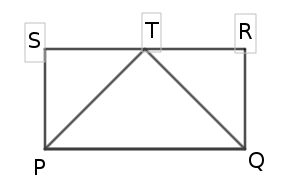a) ∠SPT = 450

b) PS = ST = 4

Get Free Study Materials + 1 Week Free Trial of BrainsPrep Class 9 English Medium Tuition

Qn 6.

In triangle ABC, AB = 6 cm, BC = 7cm, ∠B=30 . Then

a) Draw the triangle ABC.

b) Draw a right angled triangle having same area.

c) Measure the perpendicular sides

d) Find the area of the triangles

Get Free Study Materials + 1 Week Free Trial of BrainsPrep Class 9 English Medium Tuition

Qn 7.

In triangle ABC, AB = 5 cm, BC = 6cm, AC=7 cm. Draw the triangle. Then draw another triangle having the same area.

For drawing triangle  ABC

Drawing a line parellel to one

Get Free Study Materials + 1 Week Free Trial of BrainsPrep Class 9 English Medium Tuition

Qn 8.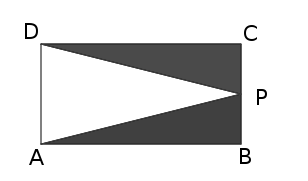In the figure ABCD is a rectangle AB=12 m and BC = 8 m. Then

Find the area of the shaded portion.

Get Free Study Materials + 1 Week Free Trial of BrainsPrep Class 9 English Medium Tuition

Qn 9.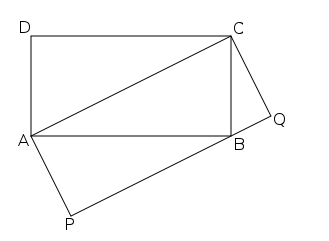In the figure ABCD is a rectangle AB=8 cm and BC = 6 cm. Then

a) What is the area of the rectangle ABCD?

b) What is the area of the rectangle APQC? Why?

c) Find the length of AP

Get Free Study Materials + 1 Week Free Trial of BrainsPrep Class 9 English Medium Tuition

Qn 10.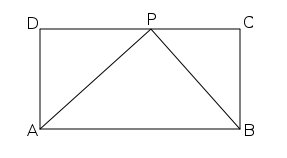In the figure ABCD is a rectangle AB=10 cm and BC = 6 cm. Then

a) What is the area of the rectangle?

b) What is the area of the triangle APB?

a) 10 x 6 = 60

b) 60/2 =